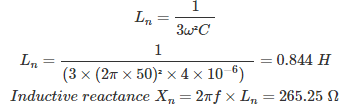# MCQs on Power Systems

##### Page 31 of 67. Go to page 1 2 3 4 5 6 7 8 9 10 11 12 13 14 15 16 17 18 19 20 21 22 23 24 25 26 27 28 29 30 31 32 33 34 35 36 37 38 39 40 41 42 43 44 45 46 47 48 49 50 51 52 53 54 55 56 57 58 59 60 61 62 63 64 65 66 67
01․ A transmission line has self and mutual impedances of 0.8 pu and 0.2 pu. Find the positive, negative and zero sequence impedances respectively?
0.6, 0.8 and 1.2 pu
0.6, 0.6 and 1.2 pu
0.8, 0.8 and 1.2 pu
0.8, 0.6 and 1.2 pu

Zs = impedance due to self inductance Zm = impedance due to mutual inductance Zero sequence impedance Z0 = Zs + 2 Zm = 0.8 + 2×0.2 = 1.2 pu Positive sequence impedance Z1 = Negative sequence impedance Z2 = Zs - Zm = 0.8 - 0.2 = 0.6 pu

02․ Positive, negative and zero sequence impedances of line are 2,2 and 5 pu respectively. Find self and mutual impedances respectively?
1 and 3 pu
3 and 1 pu
2 and 2 pu
2 and 5 pu

Zs = impedance due to self inductance Zm = impedance due to mutual inductance Zero sequence impedance Z0 = Zs + 2 Zm Positive sequence impedance Z1 = Negative sequence impedance Z2 = Zs - Zm

03․ A transmission line has self and mutual impedances are 0.8 and 0 pu respectively. Find the positive, negative and zero sequence impedances respectively?
0.6, 0.8 and 1.2 pu
0.6, 0.6 and 1.2 pu
0.6, 0.6 and 0.6 pu
0.8, 0.8 and 0.8 pu

Zs = impedance due to self inductance Zm = impedance due to mutual inductance Zero sequence impedance Z0 = Zs + 2 Zm Positive sequence impedance Z1 = Negative sequence impedance Z2 = Zs - Zm When mutual impedance is zero, Z1 = Z2 = Z0 = Zs = 0.8 pu

04․ For symmetrical network, the neutral current is
zero
infinity
maximum
any of the above

For a symmetrical network only the positive sequence component are present. Neutral current IN = IR + IY + IB Where IR, IY and IB are currents passing through three phases. Neutral current IN = 3 IR0 For symmetrical network IR1 ≠ 0 IR2 = IR0 = 0 Therefore, neutral current IN = 0 for symmetrical network.

05․ When reactance grounding is used in network, the voltage between neutral and ground is
IN × Xn
IR0 × Xn
3×IR0 × Xn
both 1 and 3

Neutral current IN = IR + IY + IB Where IR, IY and IB are currents passing through three phases. Neutral current IN = 3 IR0 When reactance grounding is used in network, the voltage between neutral and ground is VNG = IN × Xn = 3×IR0 × Xn

06․ The value of inductance used for compensation of arcing grounds is
3ω²C
1/3ω²C
3/ω²C
ω²C/3

In case of ground faults LG and LLG, arcing grounds are produced, this will cause charging current of the capacitor increase and hence voltage of the healthy phases are raised to dangerous limits, at which insulation failure of healthy phases occurs. To compensate this effect an inductive coil called 'Peterson coil' is connected between ground and the neutral. The value of inductance used for compensation of arcing grounds = 1/3ω²C

07․ The ground capacitance existing between transmission line and ground is 4µF. Find the inductive reactance of the peterson coil for compensation of arcing grounds?
0.844 Ω
855.26 Ω
265.25 Ω
625.35 Ω

The value of inductance used for compensation of arcing grounds08․ Earth fault relay uses the which of the following sequence currents?
positive sequence
negative sequence
zero sequence
any of the above

In case of unsymmetrical faults like LG, LLG the fault current pass through ground to the neutral and providing a relay between the neutral and ground, the zero sequence currents are passing through this relay for protection of electrical equipments like generator, transformer etc. This relay is called earth fault relay or ground fault relay.

09․ Which of the following grounding is not practically preferred for protection of equipments?
reactance grounding
resistance grounding
isolated grounding
all of the above

In case of unsymmetrical faults like LG, LLG the fault current pass through ground to the neutral and providing a relay between the neutral and ground, the zero sequence currents are passing through this relay for protection of electrical equipments like generator, transformer etc. This relay is called earth fault relay or ground fault relay. For isolated grounding, neutral current does not flow, so that zero sequence current is zero. Therefore, isolated grounding is not practically preferred for protection of equipments.

10․ The positive, negative and zero sequence impedances of a transmission line are 0.5,0.5 and 1.1 pu respectively. The self (Zs) and mutual (Zm) impedances of the line will be given by
Zs = 0.7 pu, Zm =0.2 pu
Zs = 0.5 pu, Zm =0.6 pu
Zs = 0.6 pu, Zm =0.5 pu
Zs = 0.2 pu, Zm =0.7 pu

Zero sequence impedance Z0 = Zs + 2 Zm Positive sequence impedance Z1 = Negative sequence impedance Z2 = Zs - Zm

<<<2930313233>>>